Word2Vec Explained Easily

Machine learning models do not understand text. Text needs to be converted into a numerical form to be fed into your models. There are various techniques for achieving this such as One Hot Encoding. The problem with One-Hot Encoding is that context is lost in the resulting vector. Word embeddings deal much better with maintaining context information in the resulting vector. In this article we will give you an easy introduction into Word2Vec.

What are Word Embeddings Anyways

Word Embedding is a set of language modeling techniques for mapping words to a vector of numbers. It’s just a fancy way of saying a numeric vector represents a word.

If you are new to this I suggest you start by learning about one-hot encoding, a basic method to generate word embeddings.

What is Word2Vec

Word2Vec was developed at Google by Tomas Mikolov, et al. and uses Neural Networks to learn word embeddings.

The beauty with word2vec is that the vectors are learned by understanding the context in which words appear. The result are vectors in which words with similar meaning end up with a similar numerical representation.

This is a big deal and greatly improve machine learning models that utilize text as input.

For example, in a regular one-hot encoded Vector, all words end up with the same distance between each other, even though there meanings are completely different. In other words, information is lost in the encoding.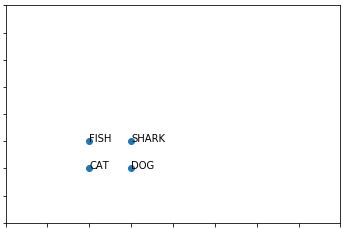With word embeddings methods such as Word2Vec, the resulting vector does a better job at maintaining context. For instance, cat and dog are more similar than fish and shark. This extra information makes a big difference in your machine learning algorithms.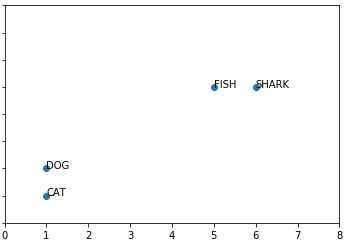How is the context learned and subsequently encoded into a vector? This is a great question and thankfully Tomas Mikolov was able to come up with a clever solution.

To understand this better you need to learn about CBOW and Skip-Gram.

CBOW vs Skip Gram

Word2Vec is composed of two different learning models, CBOW and Skip-Gram. CBOW stands for Continuous Bag of Words model.

Continuous Bag of Words (CBOW) model can be thought as learning word embeddings by training a model to predict a word given its context.

Skip-Gram Model is the opposite, learning word embeddings by training a model to predict context given a word.

Take a look at the below image.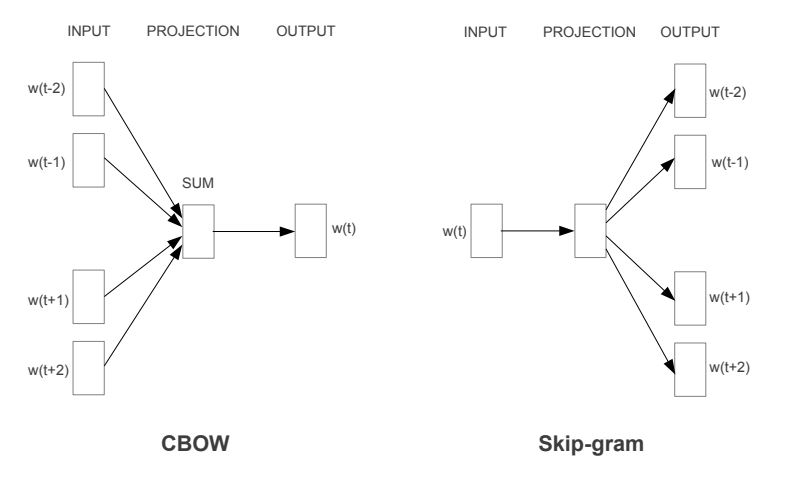Both models are built using 3 layer neural network with an input layer, hidden layer and output layer. They are in essence opposites of one another in the way the input and outputs are represented.

Other than that, for these two models the word embedding is the hidden layer of the model. You can toss out the output layer, the hidden layer is what you care about!

Skip-Gram Learning Model

Let’s dive deeper in the Skip-Gram model to better understand how they work. Remember in the Skip-Gram learning model input is a one-hot encoded word and the output is the word’s context. What is context? We will get to that now.

Imagine your vocabulary consisted of only the following 5 words and are currently inputting into your skip-gram model the word w(4) royal.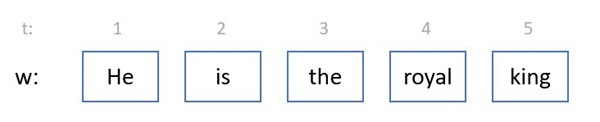Window Size defines how many words before and after the target word will be used as context, typically a Window Size is 5.

Using a window size of 1 the input pairs for training on w(4) royal would be:

• (royal, the)
• (royal, king)

Using a window size of 2 the input pairs for training on w(4) royal would be:

• (royal, the)
• (royal, king)
• (royal, is)

In this sense, a vanilla explanations for context is that context are words that usually appear with one another.

In a trained skip-gram model, by inputting the word “Royal”, the context would be predicted to be the words “The” and “King” given by the model’s output layer which contains a probability distribution of each word in the vocabulary when provided a word.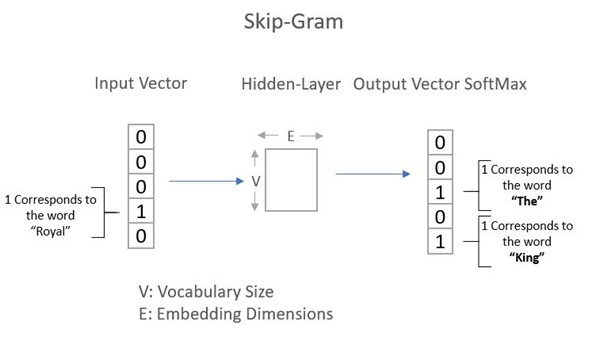Skip-Gram Model Architecture

In Skip-Gram, the input layer consists of your vocabulary a  (R x V) vector in which V=Vocabulary Size and R is the number of training samples. Each word in your vocabulary is represented by a one-hot encoded vector.

This input vector then goes through a hidden layer vector (V x E) in which E = Embedding Dimensions or Features you are trying to learn. The output layer is a vector (R x V). In the output layer, the vector holds a probability for each word in your vocabulary for the given word input. Softmax is applied to this layer.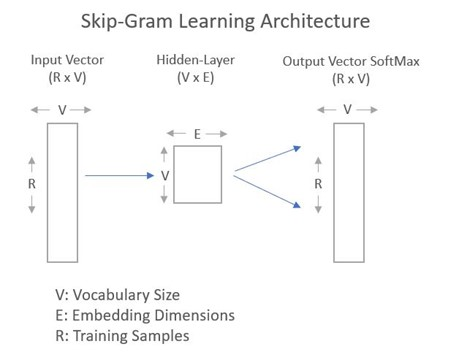The word embedding is the Hidden-Layer a vector (V x E).

For a given word this vector is a (1 x E) vector. In, essence you can think about the hidden layer as a lookup table. When you multiply the one hot encoded vector for a given word times the hidden layer vector, the result is the word embedding.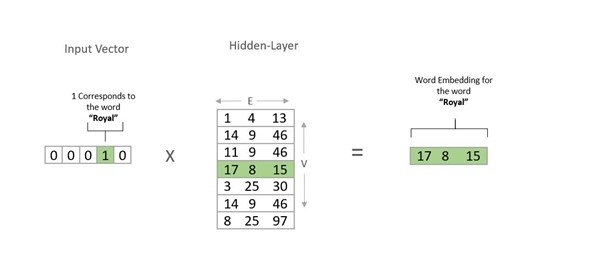Once all is over and done, words that appear under similar contexts end up having a similar vector representation. Amazing right! Using Word2Vec greatly improves your machine learning models.

Conclusion

You how now a better understanding of word embeddings and are familiar with the concepts of Word2Vec. You understand the difference between Skip-Gram and CBOW and know what a Window Size is. You also have intuition into how the word embeddings are created and that the Hidden Layer is a giant lookup table for the word embeddings. In my next article we will build train word2vec using tensorflow. Stay tuned.